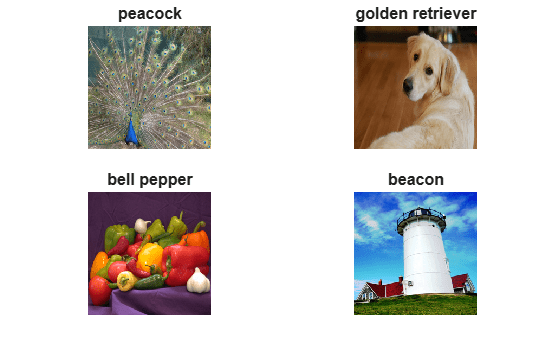# Inference Comparison Between ONNX and Imported Networks for Image Classification

This example shows how to compare the inference (prediction) results of an ONNX™ model and the imported MATLAB® network for an image classification task. First, use the network for prediction in ONNX and save the prediction results. Then, import the network in MATLAB using the `importNetworkFromONNX` function and predict the classification outputs for the same images used to predict in ONNX.

You can also use the workflow in this example to compare a MATLAB deep learning network and the exported model into ONNX. Use `exportONNXNetwork` to export a MATLAB network to the ONNX model format.

### Create ONNX Model and Image Data Set

Generate an ONNX model of the `squeezenet` convolutional neural network.

```squeezeNet = squeezenet; exportONNXNetwork(squeezeNet,"squeezeNet.onnx");```

Create the image data set.

```Im1 = imresize(imread("peacock.jpg"),[227 227]); Im2 = imresize(imread("sherlock.jpg"),[227 227]); Im3 = imresize(imread("peppers.png"),[227 227]); Im4 = imresize(imread("lighthouse.png"),[227 227]); X = cat(4,Im1,Im2,Im3,Im4);```

Create the data set that the ONNX model uses for prediction. Permute the 2-D image data from the Deep Learning Toolbox™ ordering (`HWCN`) to the ONNX ordering (`NCHW`), where `H`, `W`, and `C` are the height, width, and number of channels of the images, respectively, and `N` is the number of images.

```X_onnx = single(X); X_onnx = permute(X_onnx,[4,3,1,2]);```

Save the data set to a MAT file.

```filename = "TestIms.mat"; save(filename,"X")```

### Inference with ONNX Network

Load a pretrained ONNX network for image classification in Python® and classify new images.

Import libraries.

```import onnxruntime as rt import scipy.io as sio ```

Load the image data from `TestIms.mat`.

```data = sio.loadmat("TestIms.mat") X = data["X_onnx"] ```

```sess = rt.InferenceSession("squeezeNet.onnx") input_name = sess.get_inputs().name ```

Classify new images.

```scores = sess.run(None,{input_name:X}) ```

Save the classification scores in the MAT file `ONNXData.mat`.

```sio.savemat("ONNXData.mat", {"scores_onnx":scores}) ```

### Inference with Imported Network

Import the pretrained ONNX network into MATLAB using `importNetworkFromONNX` and classify the same images as with the ONNX network.

Specify the class names.

`ClassNames = squeezeNet.Layers(end).Classes;`

Import the pretrained `squeezeNet.onnx` model and specify the classes. `importNetworkFromONNX` imports the network as a `dlnetwork` object.

`net = importNetworkFromONNX("squeezeNet.onnx")`
```net = dlnetwork with properties: Layers: [70x1 nnet.cnn.layer.Layer] Connections: [77x2 table] Learnables: [52x3 table] State: [0x3 table] InputNames: {'data'} OutputNames: {'probOutput'} Initialized: 1 View summary with summary. ```

Predict classification scores by using the `predict` function. The predicted class label for each observation corresponds to the class with the highest score.

```scores_dlt = predict(net,single(X)); [~,ind_dlt] = max(scores_dlt,[],2); labels_dlt = ClassNames(ind_dlt);```

For this example, the data `X` is in the correct ordering. Note that if the image data `X` is in ONNX dimension ordering, you must convert `X` to the Deep Learning Toolbox ordering by entering `X = permute(X,[3,4,2,1])`.

Display the sequence of images and the classification results.

```t = tiledlayout(2,2); for i = 1:size(X,4) nexttile imshow(X(:,:,:,i)) title([ClassNames(labels_dlt(i))],FontSize=12) end t.TileSpacing = "compact"; t.Padding = "compact";```### Compare Accuracy

Load the ONNX network scores from `ONNXData.mat`, attached to this example as a supporting file. To access `ONNXData.zip`, open the example in Live Editor.

`load("ONNXData.mat")`

Compare the inference results (classification scores) of the ONNX network (`scores_onnx`) and the imported network (`scores_dlt`).

`diff = max(abs(scores_dlt-squeeze(scores_onnx)),[],"all")`
```diff = single 4.3511e-06 ```

The difference between inference results is negligible, which strongly indicates that the ONNX network and the imported network are the same.

As a secondary check, you can compare the classification labels. First, compute the class labels predicted by the ONNX network. Then, compare the labels predicted by the ONNX network and the imported network.

```[~,ind] = max(squeeze(scores_onnx),[],2); labels_onnx = categorical(ClassNames(ind))```
```labels_onnx = 4x1 categorical peacock golden retriever bell pepper beacon ```
`isequal(labels_dlt,labels_onnx)`
```ans = logical 1 ```

The labels are the same, which indicates that the two networks are the same.